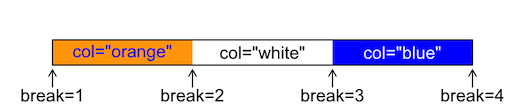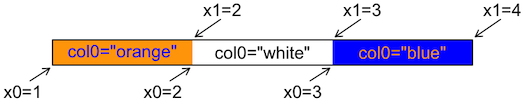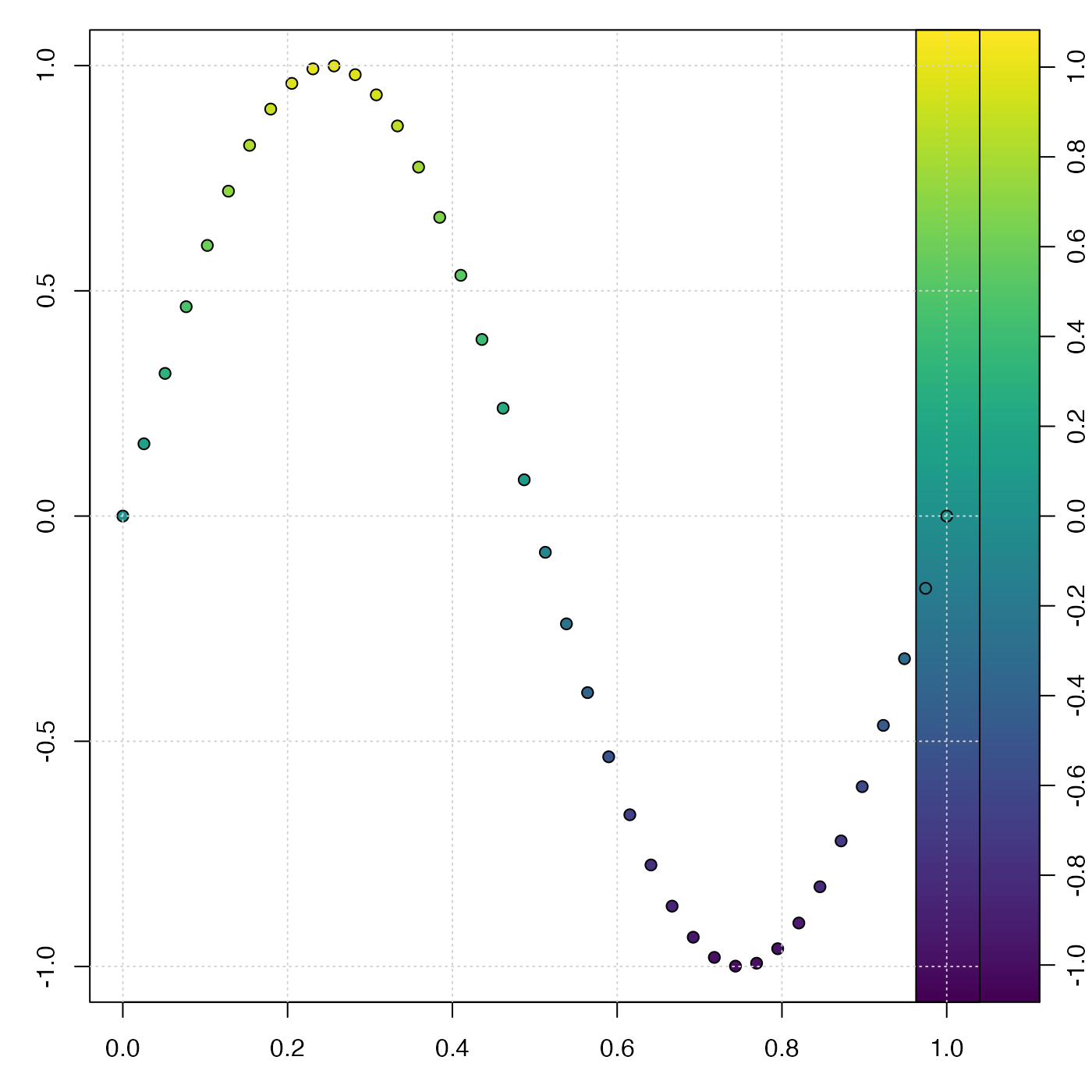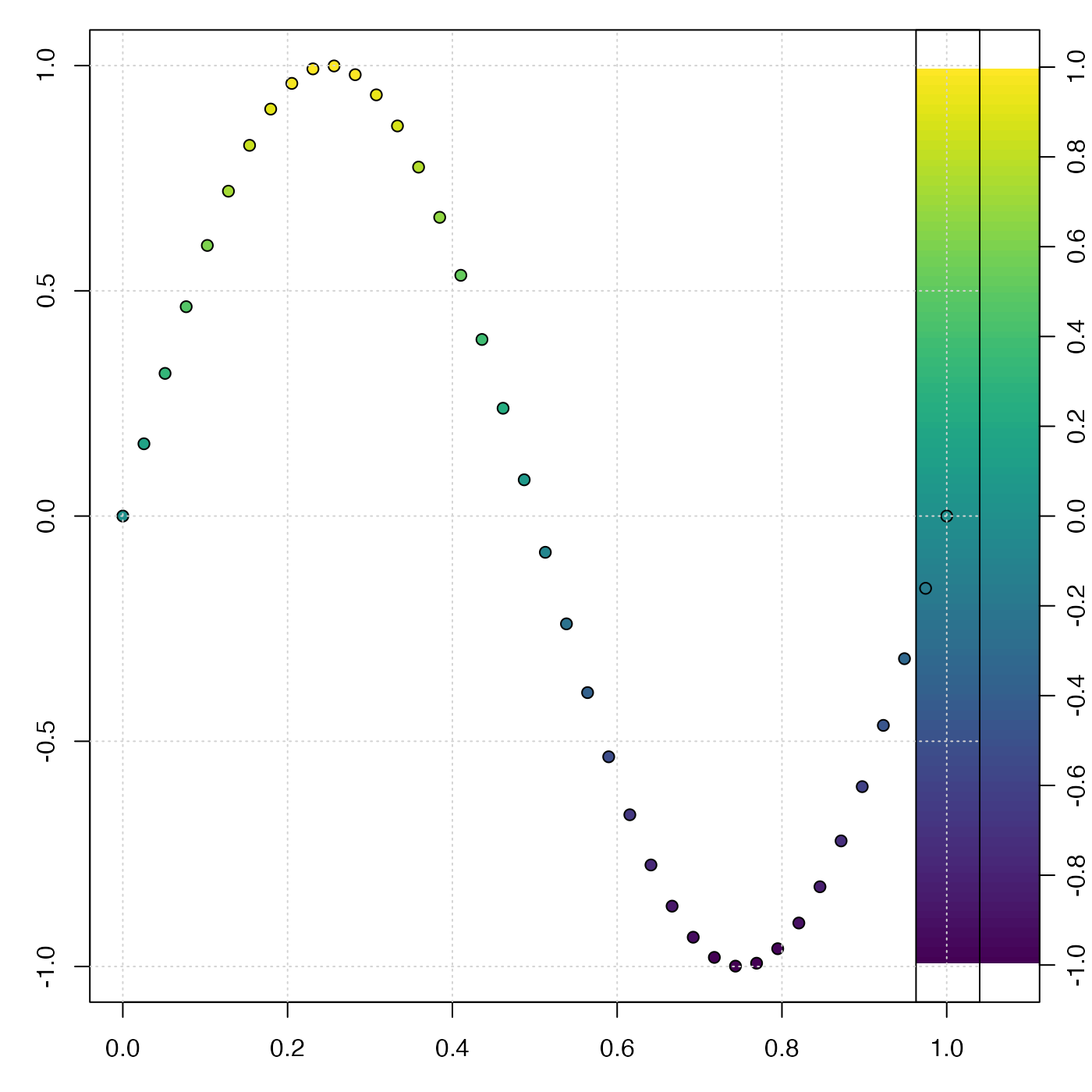Create a mapping between numeric values and colors, for use in palettes and plots. The return value can be used in various ways, including colorizing points on scattergraphs, controlling images created by image() or imagep(), drawing palettes with drawPalette(), etc.

colormap(
z = NULL,
zlim,
zclip = FALSE,
breaks,
col = oceColorsViridis,
name,
x0,
x1,
col0,
col1,
blend = 0,
missingColor,
debug = getOption("oceDebug")
)

## Arguments

z

an optional vector or other set of numerical values to be examined. If z is given, the return value will contain an item named zcol that will be a vector of the same length as z, containing a color for each point. If z is not given, zcol will contain just one item, the color "black".

zlim

optional vector containing two numbers that specify the z limits for the color scale. This can only be provided in cases A and B, as defined in “Details”. For case A, if zlim is not provided, then it is inferred by using rangeExtended() on breaks, if that is provided, or from z otherwise. Also, in case A, it is an error to provide both zlim and breaks, unless the latter is of length 1, meaning the number of subdivisions to use within the range set by zlim. In case B, zlim is inferred from using rangeExtended() on c(x0,x1). In case C, providing zlim yields an error message, because it makes no sense in the context of a named, predefined color scheme.

zclip

logical, with TRUE indicating that z values outside the range of zlim or breaks should be painted with missingColor and FALSE indicating that these values should be painted with the nearest in-range color.

breaks

an optional indication of break points between color levels (see image()). If this is provided, the arguments name through blend are all ignored (see “Details”). If it is provided, then it may either be a vector of break points, or a single number indicating the desired number of break points to be computed with pretty(z,breaks). In either case of non-missing breaks, the resultant break points must number 1 plus the number of colors (see col).

col

either a vector of colors or a function taking a numerical value as its single argument and returning a vector of colors. Prior to 2021-02-08, the default for col was oceColorsJet, but it was switched to oceColorsViridis on that date. The value of col is ignored if name is provided, or if x0 through col1 are provided.

name

an optional string naming a built-in colormap (one of "gmt_relief", "gmt_ocean", "gmt_globe" or "gmt_gebco") or the name of a file or URL that contains a color map specification in GMT format. If name is given, then it is passed to colormapGMT(), which creates the colormap. Note that the colormap thus created has a fixed relationship between value and color, and zlim, only other argument that is examined is z (which may be used so that zcol will be defined in the return value), and warnings are issued if some irrelevant arguments are provided.

x0, x1, col0, col1

Vectors that specify a color map. They must all be the same length, with x0 and x1 being numerical values, and col0 and col1 being colors. The colors may be strings (e.g. "red") or colors as defined by rgb() or hsv().

blend

a number indicating how to blend colors within each band. This is ignored except when x0 through col1 are supplied. A value of 0 means to use col0[i] through the interval x0[i] to x1[i]. A value of 1 means to use col1[i] in that interval. A value between 0 and 1 means to blend between the two colors according to the stated fraction. Values exceeding 1 are an error at present, but there is a plan to use this to indicate sub-intervals, so a smooth palette can be created from a few colors.

missingColor

color to use for missing values. This cannot be provided if name is also provided (case C), because named schemes have pre-defined colors. For other cases, missingColor defaults to "gray", if it is not provided as an argument.

debug

a flag that turns on debugging. Set to 1 to get a moderate amount of debugging information, or to 2 to get more.

## Value

a list containing the following (not necessarily in this order)

• zcol, a vector of colors for z, if z was provided, otherwise "black"

• zlim, a two-element vector suitable as the argument of the same name supplied to image() or imagep()

• breaks and col, vectors of breakpoints and colors, suitable as the same-named arguments to image() or imagep()

• zclip the provided value of zclip.

• x0 and x1, numerical vectors of the sides of color intervals, and col0 and col1, vectors of corresponding colors. The meaning is the same as on input. The purpose of returning these four vectors is to permit users to alter color mapping, as in example 3 in “Examples”.

• missingColor, a color that could be used to specify missing values, e.g. as the same-named argument to imagep().

• colfunction, a univariate function that returns a vector of colors, given a vector of z values; see Example 6.

## Details

colormap can be used in a variety of ways, including the following.

• Case A. Supply some combination of arguments that is sufficient to define a mapping of value to color, without providing x0, col0, x1 or col1 (see case B for these), or providing name (see Case C). There are several ways to do this. One approach is to supply z but no other argument, in which case zlim, and breaks will be determined from z, and the default col will be used. Another approach is to specify breaks and col together, in the same way as they might be specified for the base R function image(). It is also possible to supply only zlim, in which case breaks is inferred from that value. The figure below explains the (breaks, col) method of specifying a color mapping. Note that there must be one more break than color. This is the method used by e.g. [image()].• Case B. Supply x0, col0, x1, and col1, but not zlim, breaks, col or name. The x0, col0, x1 and col1 values specify a value-color mapping that is similar to that used for GMT color maps. The method works by using seq() to interpolate between the elements of the x0 vector. The same is done for x1. Similarly, colorRampPalette() is used to interpolate between the colors in the col0 vector, and the same is done for col1. The figure above explains the (x0, x1, col0, col1) method of specifying a color mapping. Note that the each of the items has the same length. The case of blend=0, which has color col0[i] between x0[i] and x1[i], is illustrated below.• Case C. Supply name and possibly also z, but not zlim, breaks, col, x0, col0, x1 or col1. The name may be the name of a pre-defined color palette ("gmt_relief", "gmt_ocean", "gmt_globe" or "gmt_gebco"), or it may be the name of a file (or URL pointing to a file) that contains a color map in the GMT format (see “References”). If z is supplied along with name, then zcol will be set up in the return value, e.g. for use in colorizing points. Another method for finding colors for data points is to use the colfunction() function in the return value.

Other things related to colors: colormapGMT(), oceColors9B(), oceColorsCDOM(), oceColorsChlorophyll(), oceColorsClosure(), oceColorsDensity(), oceColorsFreesurface(), oceColorsGebco(), oceColorsJet(), oceColorsOxygen(), oceColorsPAR(), oceColorsPalette(), oceColorsPhase(), oceColorsSalinity(), oceColorsTemperature(), oceColorsTurbidity(), oceColorsTurbo(), oceColorsTwo(), oceColorsVelocity(), oceColorsViridis(), oceColorsVorticity(), ocecolors

Dan Kelley

## Examples

library(oce)
# Example 1. color scheme for points on xy plot
x <- seq(0, 1, length.out=40)
y <- sin(2 * pi * x)
par(mar=c(3, 3, 1, 1))
mar <- par('mar') # prevent margin creep by drawPalette()
# First, default breaks
c <- colormap(y)
drawPalette(c$zlim, col=c$col, breaks=c$breaks) plot(x, y, bg=c$zcol, pch=21, cex=1)
grid()par(mar=mar)
# Second, 100 breaks, yielding a smoother palette
c <- colormap(y, breaks=100)
drawPalette(c$zlim, col=c$col, breaks=c$breaks) plot(x, y, bg=c$zcol, pch=21, cex=1)
grid()par(mar=mar)

if (FALSE) {
# Example 2. topographic image with a standard color scheme
par(mfrow=c(1,1))
data(topoWorld)
cm <- colormap(name="gmt_globe")
imagep(topoWorld, breaks=cm$breaks, col=cm$col)

# Example 3. topographic image with modified colors,
# black for depths below 4km.
cm <- colormap(name="gmt_globe")
deep <- cm$x0 < -4000 cm$col0[deep] <- 'black'
cm$col1[deep] <- 'black' cm <- colormap(x0=cm$x0, x1=cm$x1, col0=cm$col0, col1=cm$col1) imagep(topoWorld, breaks=cm$breaks, col=cm$col) # Example 4. image of world topography with water colorized # smoothly from violet at 8km depth to blue # at 4km depth, then blending in 0.5km increments # to white at the coast, with tan for land. cm <- colormap(x0=c(-8000, -4000, 0, 100), x1=c(-4000, 0, 100, 5000), col0=c("violet","blue","white","tan"), col1=c("blue","white","tan","yellow")) lon <- topoWorld[['longitude']] lat <- topoWorld[['latitude']] z <- topoWorld[['z']] imagep(lon, lat, z, breaks=cm$breaks, col=cm$col) contour(lon, lat, z, levels=0, add=TRUE) # Example 5. visualize GMT style color map cm <- colormap(name="gmt_globe", debug=4) plot(seq_along(cm$x0), cm$x0, pch=21, bg=cm$col0)
grid()
points(seq_along(cm$x1), cm$x1, pch=21, bg=cm$col1) # Example 6. colfunction cm <- colormap(c(0, 1)) x <- 1:10 y <- (x - 5.5)^2 z <- seq(0, 1, length.out=length(x)) drawPalette(colormap=cm) plot(x, y, pch=21, bg=cm$colfunction(z), cex=3)
}